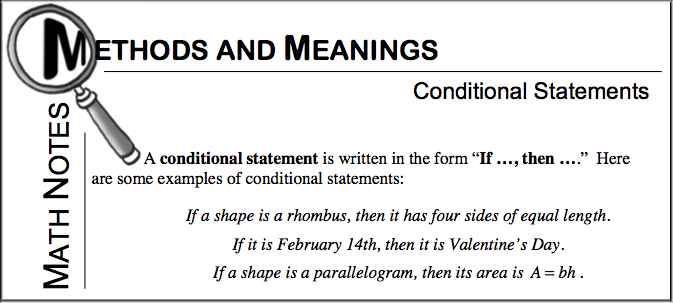Home > CCG > Chapter 7 > Lesson 7.3.3 > Problem7-143

7-143.

Examine the arrow diagram below. Homework Help ✎

Polygon is a parallelogram area of the polygon equals base times height.1. Write this conjecture as a conditional (“If, then”) statement.

If a polygon is a parallelogram, then its area equals its base times its height.

2. Write the converse of this conditional statement. Is the converse statement a true statement?

If a polygon is a triangle, then its area equals one half its base times its height.

3. Write a similar conjecture about triangles. Write it once as a conditional statement and once as an arrow diagram.

4. Write the converse of your triangle conjecture from part (c). Is the converse statement true?